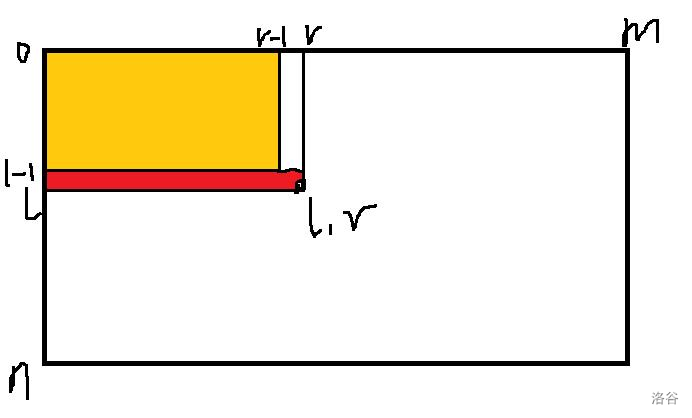5G时代下一个聚合的编程学习网

# P2516 [HAOI2010]最长公共子序列 题解

## Solution

(f_{l,r}) 表示第一个字符串匹配到 (l)，第二个字符串匹配到 (r)，并且子序列以 (a_l,b_r) 为结尾的最长长度。同时记录一个 (g_{l,r}) 表示出现次数。

[f_{l,r} = max{f_{i,j} + 1}(1 le i < l,1 le j < r,a_i = b_j)
]

[g_{l,r} = sum_{i=1}^{l-1}sum_{j=1}^{r-1}g_{i,j}(f_{l,r} = f_{i,j}+1)
]• 更新时候的大前提 (a_l=b_r) 还是要保留的。
• 但是在记录 `nowM``sum` 时，不管两个字符是否相等都要更新。
• 如果你想给 (f) 初始化极小值，那么在 (f_r < 0) （压维后） 时就不要更新对应的 (cnt_r) 了。

## Code

``````/*
Work by: Suzt_ilymics
Problem: 不知名屑题
Knowledge: 垃圾算法
Time: O(能过)
*/
#include<iostream>
#include<cstdio>
#include<cstring>
#include<algorithm>
#include<cmath>
#include<queue>
#define LL long long
#define orz cout<<"lkp AK IOI!"<<endl

using namespace std;
const int MAXN = 1e5+5;
const int INF = 1e9+7;
const int mod = 1e8;

int n, m;
char a, b;
int f, Max, cnt, p;

int s = 0, f = 0;
char ch = getchar();
while(!isdigit(ch))  f |= (ch == '-'), ch = getchar();
while(isdigit(ch)) s = (s << 1) + (s << 3) + ch - '0' , ch = getchar();
return f ? -s : s;
}

int main()
{
scanf("%s", a + 1); scanf("%s", b + 1);
n = strlen(a + 1), m = strlen(b + 1);
memset(f, 128, sizeof f);
memset(Max, 128, sizeof Max);
f = 0; Max = 0; cnt = 1;
for(int l = 1; l <= n; ++l) {
for(int r = 1, nowM = 0, sum = 0; r <= m; ++r) {
if(nowM < Max[r - 1]) nowM = Max[r - 1], sum = cnt[r - 1];
else if(nowM == Max[r - 1]) sum = (sum + cnt[r - 1]) % mod;
if(a[l] != b[r]) continue;
f[r] = nowM + 1;
p[r] = sum;
}
for(int r = 0; r <= m; ++r) {
if(f[r] < 0) continue;
if(Max[r] < f[r]) Max[r] = f[r], cnt[r] = p[r];
else if(Max[r] == f[r]) cnt[r] = (cnt[r] + p[r]) % mod;
p[r] = 0;
}
}
printf("%d
%d", f[m] - 1, cnt[m]);
return 0;
}
``````